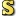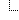﻿Complex Class
Cove - A Practical Quantum Computer Programming Framework
Complex Class
NamespacesCove.ClassicalUtilitiesComplex
A complex number class for use in simulating quantum systemsDeclaration Syntax
 C# Visual Basic Visual C++
`public class Complex : ICoveObject, ICloneable`
```Public Class Complex _
Implements ICoveObject, ICloneable```
```public ref class Complex : ICoveObject,
ICloneable```Members
 All Members Constructors Methods Fields PublicProtected InstanceStatic DeclaredInherited
IconMemberDescriptionComplex()()()
Default constuctor, creates a complex number 0 + 0i.Complex(Double)
Overload to construct a complex number with only the real portion set. (imaginary is 0)Complex(Int32)
Overload to construct a complex number with only the real portion set. (imaginary is 0)Complex(Double, Double)
Overloaded constructor to create the complex number specifiedComplex(Complex)
Construct a new complex number as a deep copy of an existing oneAbsoluteValue()()()
Return the absolute value of the complex number, defined by (a^2 + b^2)^(1/2). Since i^2 = 1, there will be no imaginary component. The absolute value is also known as the modulus. Reference: J. Stewart, Calculus, 3 ed. Pacific Grove, CA: Brooks/Cole Publishing Company, 1995.AbsoluteValueSquared()()()
Returns the square of the absolute value. Since i^2 = 1, there will be no imaginary componentAddition(Complex, Complex)
Perform addition between complex numbers. Addition between complex numbers is defined as (a + bi) + (c + di) = (a + c) + (b + d)iClone()()()
Return a deep copy of the objectDivision(Complex, Complex)
Perform division between two complex numbersEquality(Complex, Complex)
Are two complex numbers equal? Two complex numbers are equal if given complex numbers a + bi and c + di, a == c and b == d.EQUALITY_TOLERANCE
The tolerance for the equality operation. If the two numbers are being compared within this then consider them equal. This is to account for losses in accuracy because these are floating point operations. Tolerance is to 12 digits, double has a precission of 15-16 digits.Equals(Object)
Have to override the equal method
(Overrides Object.Equals(Object).)Equals(Complex)
Overloaded Equals() to compare to a Complex objectEquals(Double)
A complex number is considered equal to a double if the real part is the same as the double and the imaginary part is 0.Equals(Int32)
A complex number is considered equal to an ubt if the real part is the same as the int and the imaginary part is 0.Finalize()()()
Allows an Object to attempt to free resources and perform other cleanup operations before the Object is reclaimed by garbage collection.
(Inherited from Object.)GetComplexConjugate()()()
Get the conjugate, which is just the complex number with the sign reversed on the imaginary part.GetHashCode()()()
Returns the hash code for this object
(Overrides Object.GetHashCode()()().)GetImaginary()()()
Get the imaginary part of the complex number. This is b in the complex number a + bi.GetReal()()()
Get the real part of the complex number. This is a in the complex number a + bi.GetType()()()
Gets the Type of the current instance.
(Inherited from Object.)Implicit(Double)
Allow for implicit conversions from double to complex. This is allowed implicitly since a double is just a complex number with the imaginary part 0.Implicit(Int32)
Allow for implicit conversions from int to complex. This is allowed implicitly since an int is just a complex number with the imaginary part 0.Inequality(Complex, Complex)
Are two complex numbers not equal? Two complex numbers are equal if given complex numbers a + bi and c + di, a == c and b == d.MemberwiseClone()()()
Creates a shallow copy of the current Object.
(Inherited from Object.)Multiply(Complex, Complex)
Perform multiplication between complex numbers. Multiplication is defined as (a + bi)(c + di) = (ac - bd) + (ad + bc)iMultiply(Double, Complex)
Perform multiplication by a number. Multiplication is defined as x(a + bi) = ax + bxiMultiply(Complex, Double)
Perform multiplication by a number. Multiplication is defined as x(a + bi) = ax + bxiSet(Double)
Set the complex number. The imaginary component will be 0.Set(Double, Double)
Set the real and imaginary parts of the complex numberSetImaginary(Double)
Set only the imaginary part of the complex number and leave the imaginary part unchanged.SetReal(Double)
Set only the real part of the complex number and leave the imaginary part unchanged.SquareRoot()()()
Get the square root of this complex number.Subtraction(Complex, Complex)
Perform subtraction between two complex numbers. Subtraction is defined as (a + bi) - (c + di) = (a - c) + (b - d)iToString()()()
Return the string representation of the complex number, a + bi. For negative imaginary numbers a - bi is returned instead of a + -bi
(Overrides Object.ToString()()().)Remarks
Implements ICloneable to get deep copiesInheritance Hierarchy
 ObjectComplex

Assembly: Cove.ClassicalUtilities (Module: Cove.ClassicalUtilities) Version: 1.0.0.0 (1.0.0.0)